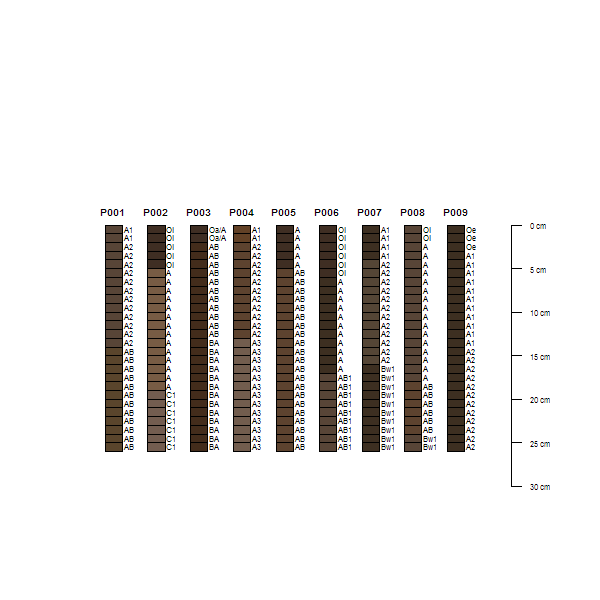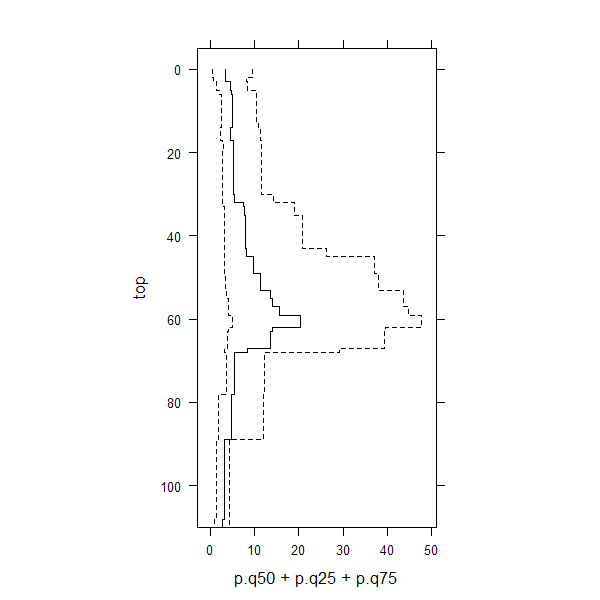sp1 {aqp} R Documentation

## Soil Profile Data Example 1

### Description

Soil profile data from Pinnacles National Monument, CA.

### Usage

`data(sp1)`

### Format

A data frame with 60 observations on the following 21 variables.

`group`

a numeric vector

`id`

a character vector

`top`

a numeric vector

`bottom`

a numeric vector

`bound_distinct`

a character vector

`bound_topography`

a character vector

`name`

a character vector

`texture`

a character vector

`prop`

a numeric vector

`structure_grade`

a character vector

`structure_size`

a character vector

`structure_type`

a character vector

`stickiness`

a character vector

`plasticity`

a character vector

`field_ph`

a numeric vector

`hue`

a character vector

`value`

a numeric vector

`chroma`

a numeric vector

### References

http://casoilresource.lawr.ucdavis.edu/

### Examples

``````data(sp1)
# convert colors from Munsell to hex-encoded RGB
sp1\$soil_color <- with(sp1, munsell2rgb(hue, value, chroma))

# promote to SoilProfileCollection
depths(sp1) <- id ~ top + bottom
site(sp1) <- ~ group

# re-sample each profile into 1 cm (thick) depth slices
# for the variables 'prop', 'name', 'soil_color'
# result is a SoilProfileCollection object
s <- slice(sp1, 0:25 ~ prop + name + soil_color)

# plot, note slices
plot(s)
````````````# aggregate all profiles along 1 cm depth slices,
# using data from column 'prop'
s1 <- slab(sp1, fm= ~ prop)

# check median & IQR
library(lattice)
xyplot(top ~ p.q50 + p.q25 + p.q75,
data=s1, type='S', horizontal=TRUE, col=1, lty=c(1,2,2),
panel=panel.superpose, ylim=c(110,-5), asp=2)
``````[Package aqp version 1.9.1 Index]